Jump to content

# Drawing Triangles, Pentagons, Stars, etc. Plugin: 2007-02-09

## Recommended Posts

I needed to draw a pentagon, but Paint.NET didn't have a tool to do that. I don't have a way to create tools, so I did the next best thing--I wrote a Codelab script.

Here are some examples of what you can create with this filter: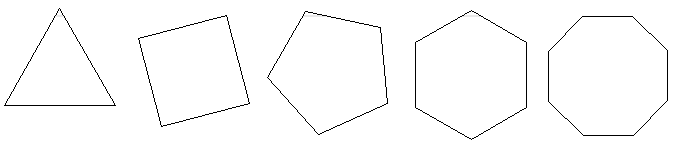If you like it, you can download the precompiled effect here: BoltBait's Plugin PackVisit this site to see step-by-step instructions how to actually use the script: http://boltbait.googlepages.com/shapes

Here is the updated Codelab script: (For you script writers... it is one of the weirdest scripts you'll ever see. Why? Because the rendering loop doesn't seem to do anything!):

```void Render(Surface dst, Surface src, Rectangle rect)
{
int rpercent = 95;        // percent of radius (1-100), default 100, slider
int verticies = 5;        // number of points (3-10?), default 5, slider
int rotation = 15;        // degrees to rotate the shape (0-359), default 0, slider
int stars = 1;            // 1=normal, 2=stars (integer, range 1 to less than verticies/2)

PdnRegion selectionRegion = EnvironmentParameters.GetSelection(src.Bounds);
Rectangle selection = this.EnvironmentParameters.GetSelection(src.Bounds).GetBoundsInt();

// Because I have to reset the destination every time
// this is reeeeeaaaaallllly slow
for(int y = rect.Top; y < rect.Bottom; y++)
{
for (int x = rect.Left; x < rect.Right; x++)
{
if (selectionRegion.IsVisible(x, y))
{
dst[x,y] = src[x,y];
}
}
}

// If this was a tool, this is where the starting nub is located
long CenterX = (long)(((selection.Right - selection.Left) / 2)+selection.Left);
long CenterY = (long)(((selection.Bottom - selection.Top) / 2)+selection.Top);

// calculate actual radius
// If this was a tool, you should calculate this from the second nub location
double r = (double)(Math.Min(CenterX-selection.Left,CenterY-selection.Top)/100.0*rpercent);

ColorBgra PrimaryColor = (ColorBgra)EnvironmentParameters.PrimaryColor; // for 2.72 use  .ForeColor;

ColorBgra CurrentPixel;
double th = 360.0/verticies;
double X1 = 0, Y1 = 0, X2 = 0, Y2 = 0;
double angle = 0;

for (int P = 0; P<=verticies; P++)
{
// Find the starting point (X1,Y1)
angle = Math.PI * ((th * P) + rotation) / 180.0;
X1 = (r * Math.Cos(angle)) + CenterX;
Y1 = (r * Math.Sin(angle)) + CenterY;
// Find next end point (X2,Y2)
angle = Math.PI * ((th * (P+stars)) + rotation) / 180.0;
X2 = (r * Math.Cos(angle)) + CenterX;
Y2 = (r * Math.Sin(angle)) + CenterY;
// Draw line from (X1,Y1) to (X2,Y2)
DrawLine((int)X1,(int)Y1,(int)X2,(int)Y2, dst, PrimaryColor, selectionRegion);
}
}

void DrawLine(int x1, int y1, int x2, int y2, Surface dst, ColorBgra LineColor, PdnRegion selectionRegion)
{
int i,gd,gm;
double roundx,roundy,xi,yi,dx,dy,x,y,l;

dx=x2-x1;
dy=y2-y1;

if(Math.Abs(dx)>Math.Abs(dy))
{
l=dx;
}
else
{
l=dy;
}

if (l<0)
{
DrawLine(x2,y2,x1,y1,dst,LineColor,selectionRegion);
}
else
{
xi=dx/l;
yi=dy/l;

x=x1;
y=y1;

if (selectionRegion.IsVisible((int)x, (int)y))
{
dst[(int)x, (int)y] = LineColor;
}

for(i=1;i<=l;i++)
{
x=x+xi;
y=y+yi;

roundx=x+0.5;
roundy=y+0.5;
if (selectionRegion.IsVisible((int)roundx, (int)roundy))
{
dst[(int)roundx, (int)roundy] = LineColor;
}
}
}
} ```

Here are some examples of stars you can create: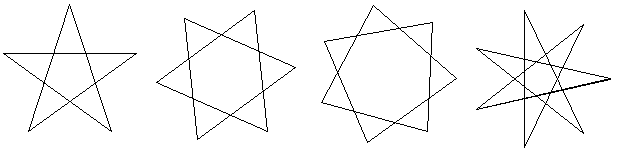Now I want to see what you can do with it!

Source Code

Since you asked, here is the VS2005 souce code: PolygonSRC.zip (Ignore the fact that I misspelled the project name.)
This should serve as a good example of how to use GDI+ drawing commands on the canvas.
This should also serve as a good example of how to use this template.

##### Share on other sites

Awesome! I'am going to creat 190371905717509 side figures! Thanks, no, realy though, awesome idea!I'm a cheerleader

I'm a Dancer

##### Share on other sites

Hi

I have got an error line 43:

``` ColorBgra PrimaryColor = (ColorBgra)EnvironmentParameters.PrimaryColor;
```

PrimaryColor isn't recognize (paint 2.72!)

I change it to

`ColorBgra PrimaryColor = (ColorBgra) 0;`

for the tests.

Try this code to have stars:

```void Render(Surface dst, Surface src, Rectangle rect)
{
int rpercent = 95;        // percent of radius (1-100), default 100, slider
int verticies = 5;        // number of points (3-10?), default 5, slider
int rotation = 0;        // degrees to rotate the shape (0-359), default 0, slider
double angfact = 2;          // angle factor to draw crossing lines, default 1, slider

PdnRegion selectionRegion = EnvironmentParameters.GetSelection(src.Bounds);
Rectangle selection = this.EnvironmentParameters.GetSelection(src.Bounds).GetBoundsInt();

// Because I have to reset the destination every time
// this is reeeeeaaaaallllly slow
for(int y = rect.Top; y < rect.Bottom; y++)
{
for (int x = rect.Left; x < rect.Right; x++)
{
if (selectionRegion.IsVisible(x, y))
{
dst[x,y] = src[x,y];
}
}
}

// If this was a tool, this is where the starting nub is located
long CenterX = (long)(((selection.Right - selection.Left) / 2)+selection.Left);
long CenterY = (long)(((selection.Bottom - selection.Top) / 2)+selection.Top);

// calculate actual radius
// If this was a tool, you should calculate this from the second nub location
double r = (double)(Math.Min(CenterX-selection.Left,CenterY-selection.Top)/100.0*rpercent);

ColorBgra PrimaryColor = (ColorBgra) 0;

ColorBgra CurrentPixel;
double th = 360.0/verticies;
double X1 = 0, Y1 = 0, X2 = 0, Y2 = 0;
double angle = 0;

// Find the first vertex
angle = Math.PI * ((th ) + rotation) / 180.0;
X1 = (r * Math.Cos(angle*angfact)) + CenterX;
Y1 = (r * Math.Sin(angle*angfact)) + CenterY;

for (int P = 0; P<=verticies; P++)
{
// Find next end point (X2,Y2)
angle = Math.PI * ((th * P) + rotation) / 180.0;
X2 = (r * Math.Cos(angle*angfact)) + CenterX;
Y2 = (r * Math.Sin(angle*angfact)) + CenterY;

// Draw line from (X1,Y1) to (X2,Y2)
DrawLine((int)X1,(int)Y1,(int)X2,(int)Y2, dst, PrimaryColor, selectionRegion);

// Get ready for the next line
X1 = X2;
Y1 = Y2;
}
}

void DrawLine(int x1, int y1, int x2, int y2, Surface dst, ColorBgra LineColor, PdnRegion selectionRegion)
{
int i;
double roundx,roundy,xi,yi,dx,dy,x,y,l;

dx=x2-x1;
dy=y2-y1;

if(Math.Abs(dx)>Math.Abs(dy))
{
l=dx;
}
else
{
l=dy;
}

if (l<0)
{
DrawLine(x2,y2,x1,y1,dst,LineColor,selectionRegion);
}
else
{
xi=dx/l;
yi=dy/l;

x=x1;
y=y1;

if (selectionRegion.IsVisible((int)x, (int)y))
{
dst[(int)x, (int)y] = LineColor;
}

for(i=1;i<=l;i++)
{
x=x+xi;
y=y+yi;

roundx=x+0.5;
roundy=y+0.5;
if (selectionRegion.IsVisible((int)roundx, (int)roundy))
{
dst[(int)roundx, (int)roundy] = LineColor;
}
}
}
}
```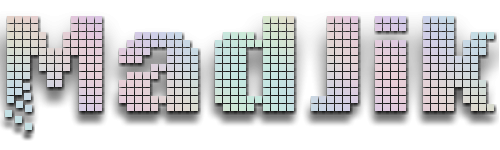My DeviantArt | My Pictorium | My Plugins | Donate via Paypal

##### Share on other sites

Test also with other values :

int verticies =9; // number of points (3-10?), default 5, slider

double angfact = 4; // angle factor to drawMy DeviantArt | My Pictorium | My Plugins | Donate via Paypal

##### Share on other sites

needs a UI... xP##### Share on other sites
Hi

I have got an error line 43:

``` ColorBgra PrimaryColor = (ColorBgra)EnvironmentParameters.PrimaryColor;
```

PrimaryColor isn't recognize (paint 2.72!)

I change it to

`ColorBgra PrimaryColor = (ColorBgra) 0;`

for the tests.

That's correct. Primary color is a 3.0 thing.

For 2.72, change the line to:

`ColorBgra PrimaryColor = (ColorBgra)EnvironmentParameters.ForeColor;`

Hey! Nice idea for creating stars. I noticed some problems with your code--it doesn't work when you choose a figure with an even number of sides and for some reason, the lines don't come out solid. I'm going to steal that idea and put it on my page. (Well, I won't steal it, per se--I'll credit you.)

##### Share on other sites

No problem! I'm also sharing ideas :wink:

I'm not c# users. But I know differents other programming languages so I can manage to adapt some code lab.

I was just trying to make your code working and add some ideas but I didn't test every cases to find out bugs (if any).My DeviantArt | My Pictorium | My Plugins | Donate via Paypal

##### Share on other sites

OK, so, taking Madjik's idea (then fixing it)...

Here is the updated Codelab script:

```void Render(Surface dst, Surface src, Rectangle rect)
{
int rpercent = 95;        // percent of radius (1-100), default 100, slider
int verticies = 5;        // number of points (3-10?), default 5, slider
int rotation = 15;        // degrees to rotate the shape (0-359), default 0, slider
int stars = 1;            // 1=normal, 2=stars (integer, range 1 to less than verticies/2)

PdnRegion selectionRegion = EnvironmentParameters.GetSelection(src.Bounds);
Rectangle selection = this.EnvironmentParameters.GetSelection(src.Bounds).GetBoundsInt();

// Because I have to reset the destination every time
// this is reeeeeaaaaallllly slow
for(int y = rect.Top; y     {
for (int x = rect.Left; x         {
if (selectionRegion.IsVisible(x, y))
{
dst[x,y] = src[x,y];
}
}
}

// If this was a tool, this is where the starting nub is located
long CenterX = (long)(((selection.Right - selection.Left) / 2)+selection.Left);
long CenterY = (long)(((selection.Bottom - selection.Top) / 2)+selection.Top);

// calculate actual radius
// If this was a tool, you should calculate this from the second nub location
double r = (double)(Math.Min(CenterX-selection.Left,CenterY-selection.Top)/100.0*rpercent);

ColorBgra PrimaryColor = (ColorBgra)EnvironmentParameters.PrimaryColor; // for 2.72 use  .ForeColor;

ColorBgra CurrentPixel;
double th = 360.0/verticies;
double X1 = 0, Y1 = 0, X2 = 0, Y2 = 0;
double angle = 0;

for (int P = 0; P    {
// Find the starting point (X1,Y1)
angle = Math.PI * ((th * P) + rotation) / 180.0;
X1 = (r * Math.Cos(angle)) + CenterX;
Y1 = (r * Math.Sin(angle)) + CenterY;
// Find next end point (X2,Y2)
angle = Math.PI * ((th * (P+stars)) + rotation) / 180.0;
X2 = (r * Math.Cos(angle)) + CenterX;
Y2 = (r * Math.Sin(angle)) + CenterY;
// Draw line from (X1,Y1) to (X2,Y2)
DrawLine((int)X1,(int)Y1,(int)X2,(int)Y2, dst, PrimaryColor, selectionRegion);
}
}

void DrawLine(int x1, int y1, int x2, int y2, Surface dst, ColorBgra LineColor, PdnRegion selectionRegion)
{
int i,gd,gm;
double roundx,roundy,xi,yi,dx,dy,x,y,l;

dx=x2-x1;
dy=y2-y1;

if(Math.Abs(dx)>Math.Abs(dy))
{
l=dx;
}
else
{
l=dy;
}

if (l   {
DrawLine(x2,y2,x1,y1,dst,LineColor,selectionRegion);
}
else
{
xi=dx/l;
yi=dy/l;

x=x1;
y=y1;

if (selectionRegion.IsVisible((int)x, (int)y))
{
dst[(int)x, (int)y] = LineColor;
}

for(i=1;i      {
x=x+xi;
y=y+yi;

roundx=x+0.5;
roundy=y+0.5;
if (selectionRegion.IsVisible((int)roundx, (int)roundy))
{
dst[(int)roundx, (int)roundy] = LineColor;
}
}
}
}```

Here are some examples of stars you can create:Now, who will put a UI on this?

##### Share on other sites

whatever happened to 'put this dll in the appropriate folder, and start using the plug-in'. all this code wearies me.

Unless one is born again, he cannot enter the kingdom of God.

Search Onion Optimisation

##### Share on other sites
whatever happened to 'put this dll in the appropriate folder, and start using the plug-in'. all this code wearies me.

So what is your question or request ? :?:My DeviantArt | My Pictorium | My Plugins | Donate via Paypal

##### Share on other sites
• 3 weeks later...

Madjik, you could put a dropdown box with the options "star" and "poligon". It's kinda stupid to have a 1-255 slider instead if sometimes nothing appears or appears a star or appears a poligon.

A brush width slider would also be nice.##### Share on other sites
A brush width slider would also be nice.

Problem with that^ is that the script does not support brush widths.##### Share on other sites

@ Trickman:

I asked for help for GUI : result near none !

I tried to adapt sources, I found standard GUI with one, two or three sliders. That's what I used for Polygones. I could give my source as well if you want to adapt it, I will look forward to see what you'll change to make it "less stupid" !

@ boilbait:

Thanks for your answer!My DeviantArt | My Pictorium | My Plugins | Donate via Paypal

##### Share on other sites

Some more explanation about "starisation" with the Polygone Effect:

Let's start with a simple one : Vertice = 7 / Stars = 1.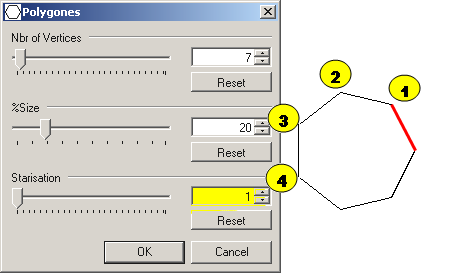When drawing the polygone the line simply goes from point 0 (not shown, sorry) to point 1 to point 2 etc...

If then we change stars to 2: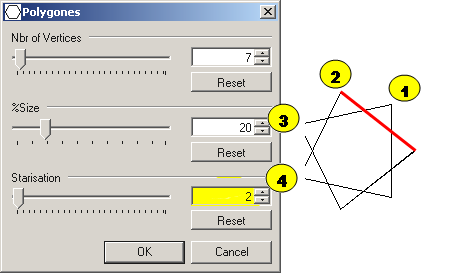The line will skip one point. The line goes from point 0 to point 2 to point 4 etc...

Then we have crossing lines that are drawing a star.

If then we change stars to 3: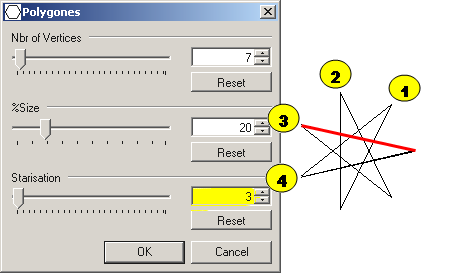The line will skip two points. The line goes from point 0 to point 3 to point 6 etc...

Then the star looks fine.

If then we change stars to 4: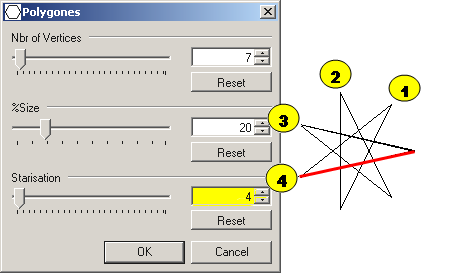The line will skip three points. The line goes from point 0 to point 4 to point 1 etc...

Then the star looks exactly as the previous one (stars=3) but it is drawn the other way.

If then we change stars to 5 then the star should look as stars=2 but it is drawn the other way.

If then we change stars to 6 then the star should look as stars=1 but it is drawn the other way.

And finaly if we set stars to 7 then all lines are going from point 0 to point 0 so we have a spot.

To have some kind of stickers you should draw a star with a lot of vertices like this example (vertice=29 stars=9)

With the magic wand select outside the star and invert the selection by Ctrl+I then use the gradient effect to fill it.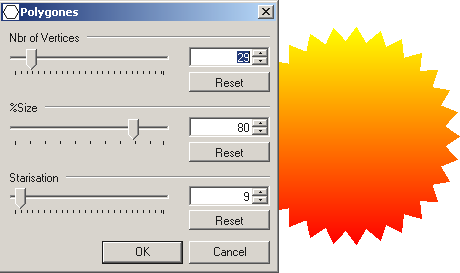My DeviantArt | My Pictorium | My Plugins | Donate via Paypal

##### Share on other sites

That looks cool! I'll have to download it.

##### Share on other sites
• 1 month later...
==> to BoltBait :

Could you change the topic title (Codelab + Plugin...)?

Sorry, this is not possible. The subject line has a limited length. This would make it too long.

##### Share on other sites

You could call it "Polygon and Theta Curves Plugin(Codelab)".

##### Share on other sites

Theta Curves isn't a term known by the "general population"Imagine:

wtf are theta curves?!?!

~~

##### Share on other sites

I can see it. Yea, people will be drawn away by the "technical" name, (well, the people that dont understand it)I'm a cheerleader

I'm a Dancer

##### Share on other sites

Could I call it Madjik's curves plugins?My DeviantArt | My Pictorium | My Plugins | Donate via Paypal

##### Share on other sites

Yes but when people think "Curves" w.r.t. image editing software, they think of this: http://www.getpaint.net/doc/3.0/en/Curves.html . Maybe replace the word "curves" with "shapes."

The Paint.NET Blog: https://blog.getpaint.net/

Donations are always appreciated! https://www.getpaint.net/donate.html

##### Share on other sites

Lol, i wish i knew how to use the

codelab.!##### Share on other sites

MadJik, could you send me the source code to the Polygon effect? I would like to look at it.

Also, I've been thinking a lot about effect submenus. I think that anything that creates something new (like drawing shapes) should be in a submenu called "Render".

Here is how I group the effects that I have written:

http://boltbait.googlepages.com/

## Join the conversation

You can post now and register later. If you have an account, sign in now to post with your account.Reply to this topic...

×   Pasted as rich text.   Paste as plain text instead

Only 75 emoji are allowed.

×   Your link has been automatically embedded.   Display as a link instead

×   Your previous content has been restored.   Clear editor

×   You cannot paste images directly. Upload or insert images from URL.

×
×

• #### Activity

• Leaderboard
×
• Create New...